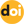The peer-reviewed scientific and technology journal. ISSN: 0204-3327

# Nodal SN method for HEX-Z-geometryhttps://doi.org/10.26583/npe.2015.3.06

### UDC: 621.039

The problem of spatial approximation for the neutron transport equation becomes very important in solving neutronics problems with coarse spatial grid, like fuel assemblies calculations of fast reactors (for example, BN-800 and BN-1200). «Weighted diamond» (WDD) schemes are the most widely adopted among the finite difference schemes for the neutrons and gamma rays transport equation. They are effective in terms of ease of implementation and computational costs. However, the problems mentioned above indicate their limitations. Diamond difference scheme (DD) has a second-order approximation (best for this type of schemes) and does not have the properties of positive and monotony. For this reason, a negative values or nonphysical oscillations appear in the solution. Step scheme (St) is monotonic and positive unlike DD, but is has only first-order accuracy. So it is inefficient for high-precision calculations.

There are negative correction algorithms and adaptive (AWDD) schemes for reducing the level of oscillation and obtaining a positive solution. However, these algorithms have a negative impact on the order of approximation, and in such cases we can obtain schemes with only 1– 2 accuracy order. In addition to adaptive schemes there is a problem of choosing the scheme’s parameters.

The obvious way out to improve the quality and accuracy of the calculation is a refinement of the grid. In the case of fast reactors spatial grid is a set of rectangular prisms with a regular hexagon at the base (HEX-Z geometry). Therefore hexagonal cells are divided into rhomboid (3 rhombus for 1 hexagon; 12 rhombus for 1 hexagon, etc.). Next we can use diamond difference scheme on a grid of rhombuses. Defects will not occur due to small cell size compared to the initial hexagonal cell. It can also be used triangular mesh.

Another approach to solving the identified problem is the development of higher order accuracy methods without the increasing number of calculation points. One of these methods is the nodal method. The basis of every nodal method is the expansion of the unknown function in the node (elementary volume with the same properties) using basic functions with subsequent calculation of expansion moments. In this article, we will focus on SN nodal method in HEXZ-geometry.

1. Ikeda H., Takeda T. A new nodal SN transport method for three-dimensional hexagonal geometry. Journal of Nuclear Science and Technology. 1994, v. 31, pp. 497-509.
2. Ikeda H., Takeda T., 3-D Neutron Transport Benchmarks. Department of Nuclear Engineering Osaka University, Japan, NEACRP L-330, 1991.
3. Yang X., Satvat N. MOCUM: A two-dimensional method of characteristics code based on constructive solid geometry and unstructured meshing for general geometries. Annals of Nuclear Energy. 2012, v. 46, pp. 20-28, 2012.
4. Tae Hyeong, Nam Zin Source projection analytic nodal SN method for hexagonal geometry. Annals of Nuclear Energy. 1996, v. 23, pp. 133-143.